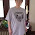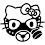## Saturday, July 16, 2016

### Katy Perry Rise Decoded

Rise in Simple Gematria Equals: 51
Demon in Simple Gematria Equals: 51

[Verse 1]
I won't just survive=69
Oh, you will see me thrive=107
Can't write my story=77
I'm beyond the archetype=104
I won't just conform=73
No matter how you shake my core=120
Cause my roots, they run deep, oh=122
69+107+77+104+73+120+122=672
Broken down..
6+9=15=6 1+0+7=8 7+7=14=5 1+0+4=5 7+3=10=1
1+2+0=3 1+2+2=5
6+8+5+5+1+3+5=33

Translation "Clinton will win, this song will play at the Olympics"
Olympics in English Gematria Equals: 672
Victory in English Gematria Equals: 672
Clinton" in the English Reduction system equals 33

[Pre-Chorus 1]
Oh, ye of so little faith=95
Don't doubt it, don't doubt it=90
Victory is in my veins=99
I know it, I know it=76
And I will not negotiate=94
I'll fight it, I'll fight it=116
I will transform=72
[Chorus]
When, when the fire's at my feet again=146
And the vultures all start circling=125
They're whispering, "you're out of time."=174
But still, I rise=58
This is no mistake, no accident=108
When you think the final nail is in; think again=195
Don't be surprised, I will still rise=143
95+90+99+76+94+116+72+146+125+174+58+108+195+143=1591
Broken Down
9+5=14=5 9+0=9 9+9=18=9 7+6=13=4 9+4=13=4 1+1+6=8 7+2=9 1+4+6=11=2 1+2+5=8 1+7+4=12=3 5+8=13=4 1+0+8=9 1+9+5=15=6 1+4+3=8
5+9+9+4+4+8+9+2+8+3+4+9+6+8=88

Translation "(I will 911 Trump)
1591
And I Will Establish My Covenant With You Neither Shall All Flesh Be Cut Off Any More By The Waters Of A Flood Neither Shall There Any More Be A Flood To Destroy The Earth in Simple Gematria Equals: 1591
Genesis 9:11
http://biblehub.com/genesis/9-11.htm
88
Trump in Simple Gematria Equals: 88

[Verse 2]
I must stay conscious=67
Through the menace and chaos=110
So I call on my angels=70
They say...=31
67+110+70+31=278
Broken down
6+7=13=4 1+1+0=2 7+0=7 3+1=4
4+2+7+4=17

Translation "The Angel Of The Bottomless Pit is my God"
278
Angel Of The Bottomless Pit in Simple Gematria Equals: 278
17
"God" in the English Reduction system equals 17

[Pre-Chorus 2]
Oh, ye of so little faith=95
Don't doubt it, don't doubt it=90
You know it, you know it=90
And you will not negotiate=101
Just fight it, just fight it=100
And be transformed=69
[Chorus]
When, when the fire's at my feet again=146
And the vultures all start circling=125
They're whispering, "you're out of time."=174
But still, I rise=58
This is no mistake, no accident=108
When you think the final nail is in; think again=195
Don't be surprised, I will still rise=143
95+90+113+90+101+100+69+146+125+174+58+108+195+143=1607
Broken down
9+5=14=5 9+0=9 1+1+3=5 9+0=9 1+0+1=2 1+0+0=1
6+9=15=6 1+4+6=11=2 1+2+5=8 1+7+4=12=3 5+8=13=4 1+0+8=9 1+9+5=15=6 1+4+3=8
5+9+5+9+2+1+6+2+8+3+4+9+6+8=77

Translation "I will 911 Jerusalem"
1607
Jeremiah Eleven Nine in Jewish Gematria Equals: 1607
Jeremiah 11:9
And the LORD said unto me, A conspiracy is found among the men of Judah, and among the inhabitants of Jerusalem.
http://biblehub.com/jeremiah/11-9.htm
MIRROR
Jeremiah 9:11
And I will make Jerusalem heaps, and a den of dragons; and I will make the cities of Judah desolate, without an inhabitant.
77
Judaism in Simple Gematria Equals: 77
"September Eleventh" in the English Reduction system equals 77

[Outro]
Don't doubt it, don't doubt=79
Oh, oh, oh, oh=56
You know it, you know it=90
Still rise=42
Just fight it, just fight it=100
Don't be surprised, I will still rise=143
79+56+90+42+100+143=510
Broken down
7+9=16=7 5+6=11=2 9+0=9 4+2=6 1+0+0=1 1+4+3=8
7+2+9+6+1+8=33
Hillary in English Gematria Equals: 510
Clinton" in the English Reduction system equals 33

Translation Signed Hillary Clinton

Really strange..
33+88+17+77+33=248
President Donald Trump in Simple Gematria Equals: 248

If anybody else see's a different translation in these numbers let me know.

1.World Emoji Day" in the English Reduction system equals 64
Synagogue of Satan" in the English Reduction system equals 64

1.Picking up on your comment there...From Katy Perry's SB Halftime performance on 2/1/15 to Election Day =646 Days.

Israel=64. Zion=64. Thelema=64. etc etc.

Katy Perry Rise =1320. Hillary Clinton =1032. Hillary Clinton Rise =223.

Katy Perry =58. Hillary =85. Looks like Katy Perry will be to Hillary what Beyonce is to Obama.

The song Rise is solely made/sung for Ritualistic reasons, but I refuse to get onboard with Hillary winning the Election. No Chance. After RISE comes FALL. Will be looking fwd to that.

Hillary For Prison....Not President.

2.Wow great work Maestro! I didn't even see these connections!
Katy Perry Rise =1320. Hillary Clinton =1032. Hillary Clinton Rise =223.

2.I like all your connections on this post, & regarding the very last one....

President Donald Trump =248. Eight Eleven Sixteen =1248. They say the 1 in front is for Fulfillment.

1.Ha, obviously I meant 11/8/16... Not 8/11.

3.I made just the opposite connection with another Katy Perry song. "Dark Horse". I connected it to Obama. When he was running in 2008 they were calling him the Dark Horse Candidate.

In the song Katy is playing a character call katy-patra who is accepting gifts from foreign leaders. If she doesn't like the gift she puts some kind of a spell on the leader. The Pharaoh gives here a gift she likes, but she still turns the Pharaoh into a dog. The dog's face looks alot like Obama's. The lap-dog of the NWO, so to speak. The United States symbolically seems like a re-do of Ancient Egypt. So with Obama. the 1st black president, would seem to be like an American Pharaoh.

Now we get to the race horse American Pharoah, which the media kept calling the "dark horse" in horse racing, tying it to the Katy Perry song, and Obama. Remember that American Pharoah won the triple crown. So I originally cast Obama as a third-term president. The Triple Crown for America's Pharaoh.

1.Note the time of my post. :-)

2.Perry is being used for the endtime narrative no doubt.

3.Stay tuned. I used to laugh at "Obama third term theorists" no more. Especially after what just happened in Turkey. Looks like Hitler's Reichstag model is back in style.

4.I don't know any more, hard to guess. Hillary is a terrible candidate, though. That will be more obvious when the campaign heats up. But it does seem like the system is coding for here.

Another possibility is that Pharaoh's third crown could be a high position in a newly activated United Nations after he leave office.

5.Oh the United Nations. Now there's a worthy decode. It would take ten people though.

6.Anything evil is possible. I don't doubt any of it for a minute... which is not to say that I'm 'on board' with this witch's crap.

4.Your decode is definitely correct. Katy Perry performed this song at the DNC: https://www.youtube.com/watch?v=df0xj-d-IGI

5.Katy Perry is a very talented musician and she is always creative and unique. Her songs sets her apart from the rest of the musicians and this is evident by the quality of the shared lyrics. I will be visiting this site to follow up on newly release songs from the musician as I look for professional writers who offer Academic Coursework Help.